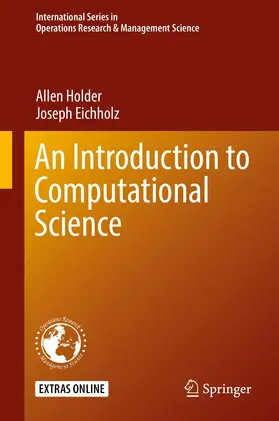# Holder / EichholzAn Introduction to Computational Science

1. Auflage 2019, Band: 278, 470 Seiten, Gebunden, Book, Format (B × H): 160 mm x 240 mm, Gewicht: 882 g Reihe: International Series in Operations Research & Management Science
ISBN: 978-3-030-15677-0
Verlag: Springer, Berlin

## Holder / EichholzAn Introduction to Computational Science

This textbook provides an introduction to the growing interdisciplinary field of computational science. It combines a foundational development of numerical methods with a variety of illustrative applications spread across numerous areas of science and engineering. The intended audience is the undergraduate who has completed introductory coursework in mathematics and computer science. Students gain computational acuity by authoring their own numerical routines and by practicing with numerical methods as they solve computational models. This education encourages students to learn the importance of answering: How expensive is a calculation, how trustworthy is a calculation, and how might we model a problem to apply a desired numerical method?The text is written in two parts. Part I provides a succinct, one-term inauguration into the primary routines on which a further study of computational science rests. The material is organized so that the transition to computational science from coursework in calculus, differential equations, and linear algebra is natural. Beyond the mathematical and computational content of Part I, students gain proficiency with elemental programming constructs and visualization, which are presented in MATLAB syntax. The focus of Part II is modeling, wherein students build computational models, compute solutions, and report their findings. The models purposely intersect numerous areas of science and engineering to demonstrate the pervasive role played by computational science.

Graduate

## Weitere Infos & Material

Chapter 1. Solving Single Variable Equations.- Chapter 2. Solving Systems of Equations.- Chapter 3. Approximation.- Chapter 4. Optimization.- Chapter 5. Ordinary Di erential Equations.- Chapter 6. Stochastic Methods & Simulation.- Chapter 7. Computing Considerations.- Chapter 8. Modeling with Matrices.- Chapter 9. Modeling with Ordinary Di erential Equations.- Chapter 10. Modeling with Delayed Di erential Equations.- Chapter 11. Partial Di erential Equations.- Chapter 12. Modeling with Optimization and Simulation.- Chapter 13. Regression Modeling.- Appendix A.

Holder, Allen
Allen Holder received his B.S. from the University of Southern Mississippi and his Ph.D. from the University of Colorado at Denver. He taught at Trinity University in San Antonio, Texas for nine years before joining Rose-Hulman. He is interested in mathematical programming and its applications in medicine, biology, and healthcare. Professor Holder's work on the optimal design of radiotherapy treatments won the 2000 Pierskalla Award, and he received Rose-Hulman's Outstanding Scholar Award in 2015. He has held several editorial positions and has published with numerous undergraduates. He is a longtime supporter of, and participant in, INFORMS.
Joseph Eichholz received his B.S. in Mathematics and Computer Science from Western Illinois University and his Ph.D. in Applied Mathematics and Computational Sciences from the University of Iowa. He is interested in numerical analysis, particularly with numerical solutions of partial differential equations and integral equations, as well as high performance computing. He has a special interest in applications associated with novel medical imaging techniques and contact mechanics.

WIR VERWENDEN COOKIES

Einige Cookies sind notwendig für den Betrieb der Seite, während andere uns helfen, Ihnen ein optimales Erlebnis unserer Webseite zu ermöglichen.